# Preparation and Properties of Compounds Questions and Answers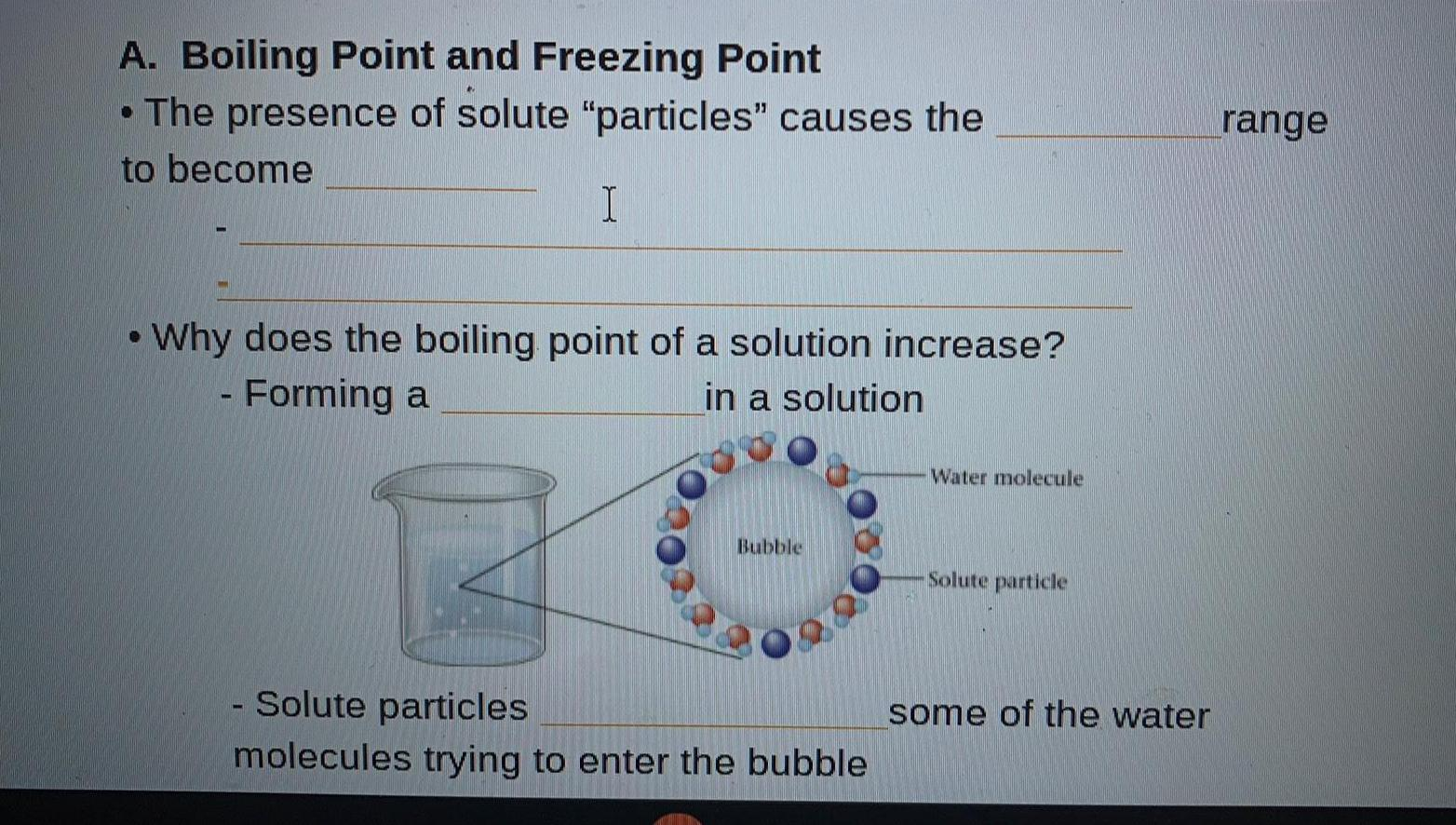Inorganic Chemistry
Preparation and Properties of Compounds
A. Boiling Point and Freezing Point • The presence of solute "particles" causes the to become__ • Why does the boiling point of a solution increase? - Forming a_____in a solution in a solution - Solute particles_____molecules trying to enter the bubble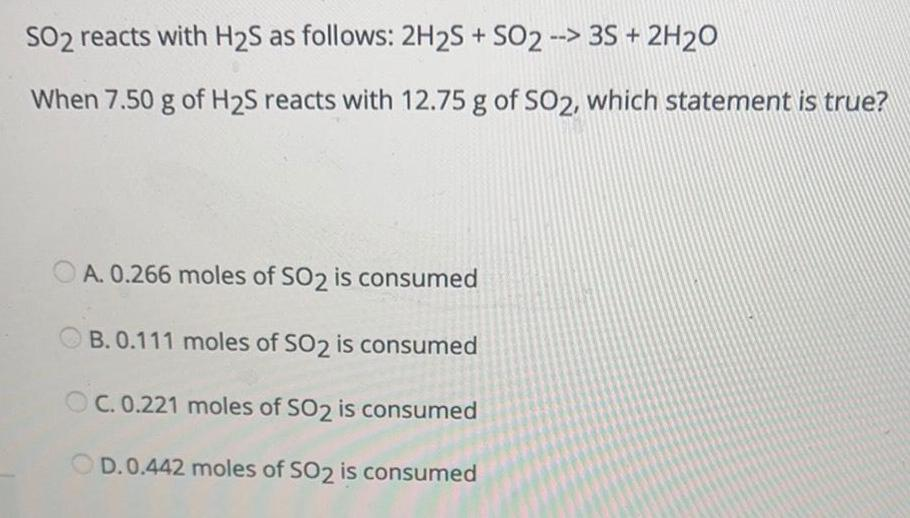Inorganic Chemistry
Preparation and Properties of Compounds
SO2 reacts with H₂S as follows: 2H2S + SO2 --> 3S + 2H2O When 7.50 g of H2S reacts with 12.75 g of SO2, which statement is true? A. 0.266 moles of SO2 is consumed B. 0.111 moles of SO2 is consumed C. 0.221 moles of SO2 is consumed D.0.442 moles of SO2 is consumed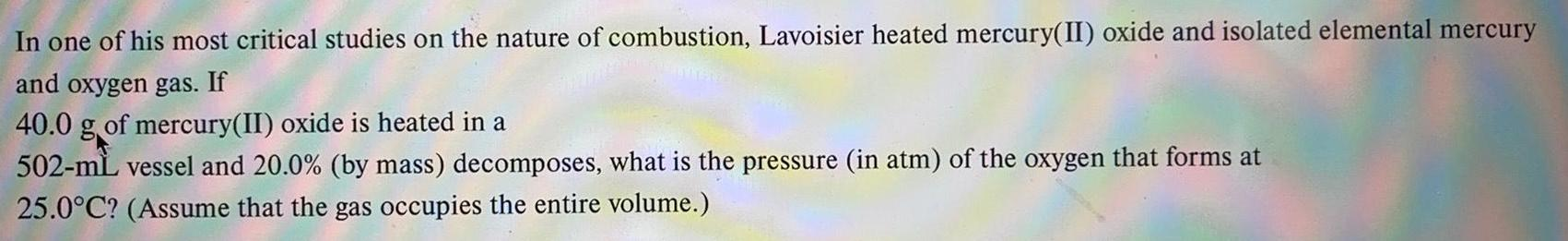Inorganic Chemistry
Preparation and Properties of Compounds
In one of his most critical studies on the nature of combustion, Lavoisier heated mercury(II) oxide and isolated elemental mercury and oxygen gas. If40.0 g of mercury(II) oxide is heated in a 502-mL vessel and 20.0% (by mass) decomposes, what is the pressure (in atm) of the oxygen that forms at 25.0°C? (Assume that the gas occupies the entire volume.)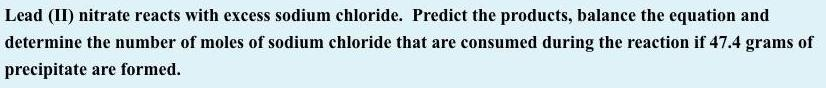Inorganic Chemistry
Preparation and Properties of Compounds
Lead (II) nitrate reacts with excess sodium chloride. Predict the products, balance the equation and determine the number of moles of sodium chloride that are consumed during the reaction if 47.4 grams of precipitate are formed.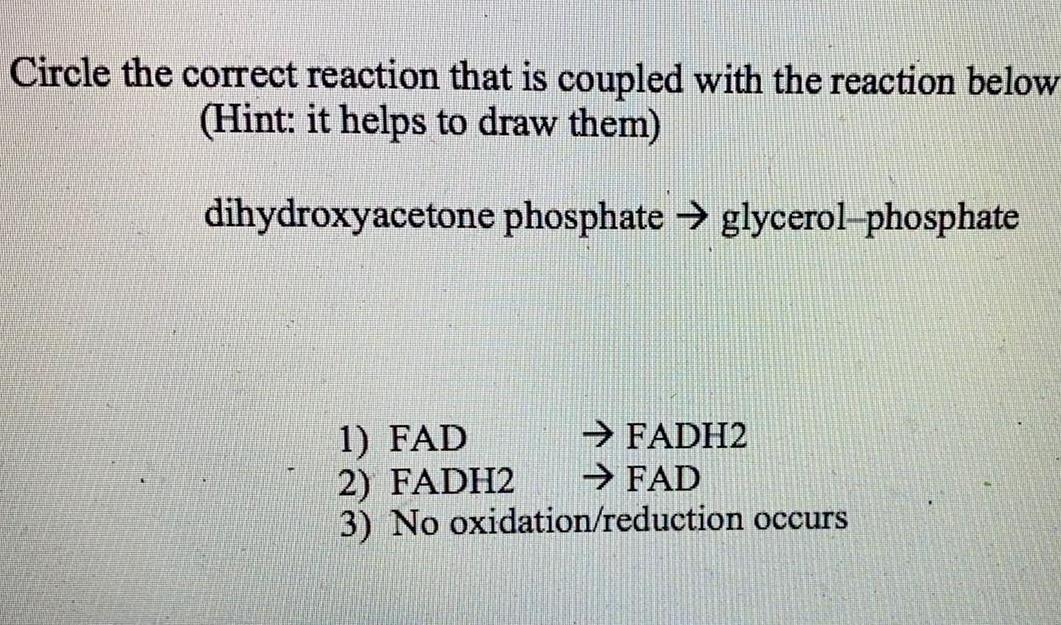Inorganic Chemistry
Preparation and Properties of Compounds
Circle the correct reaction that is coupled with the reaction below (Hint: it helps to draw them) dihydroxyacetone phosphate → glycerol-phosphate 1) FAD → FADH2 2) FADH2 → FAD 3) No oxidation/reduction occurs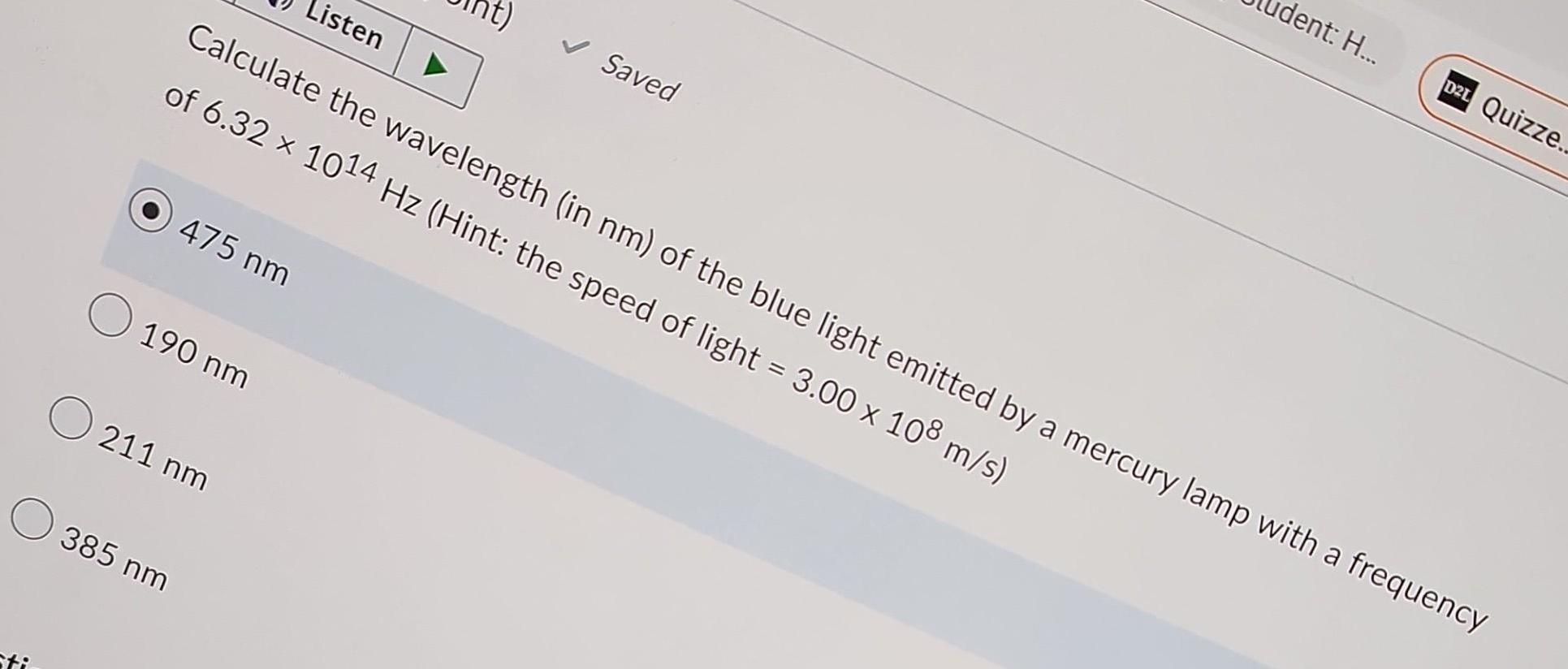Inorganic Chemistry
Preparation and Properties of Compounds
Calculate the wavelength (in nm) of the blue light emitted by a mercury lamp with a frequency of 6.32 × 10^14 Hz (Hint: the speed of light = 3.00 x 10^8 m/s) 475 nm 190 nm 211 nm 385 nm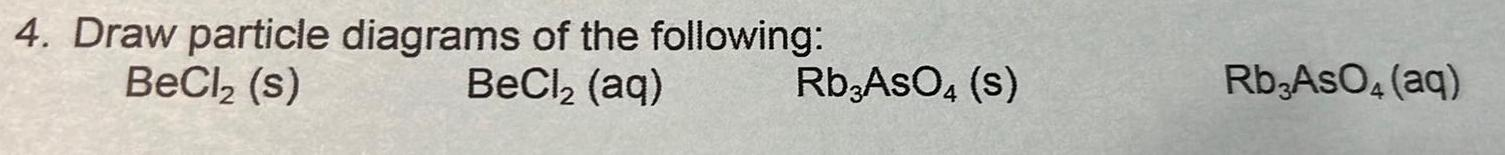Inorganic Chemistry
Preparation and Properties of Compounds
Draw particle diagrams of the following: BeCl₂ (s) BeCl₂ (aq) Rb3AsO4(s) Rb3AsO4 (aq)Inorganic Chemistry
Preparation and Properties of Compounds
Elements of "A Warn Path" seem exaggerated and strange. What is the literary term for this genre that Welty's writing often fits into? Realism Romanticism Southern Gothic Mysticism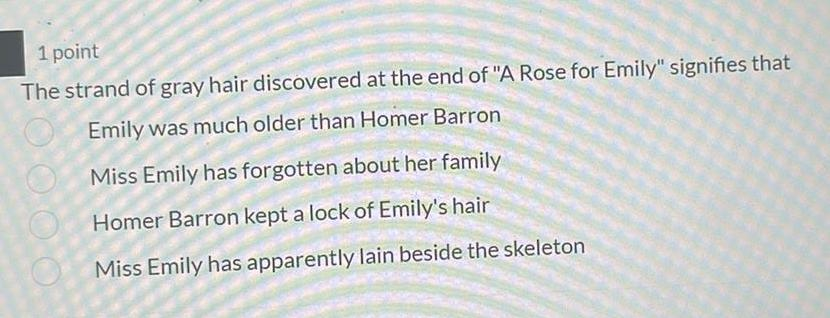Inorganic Chemistry
Preparation and Properties of Compounds
The strand of gray hair discovered at the end of "A Rose for Emily" signifies that Emily was much older than Homer Barron Miss Emily has forgotten about her family Homer Barron kept a lock of Emily's hair Miss Emily has apparently lain beside the skeleton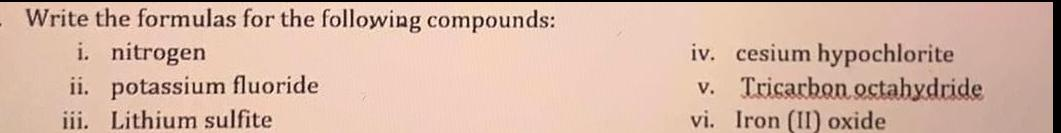Inorganic Chemistry
Preparation and Properties of Compounds
Write the formulas for the following compounds: i. nitrogen ii. potassium fluoride iii. Lithium sulfite iv. cesium hypochlorite v. Tricarbon octahydride vi. Iron (II) oxide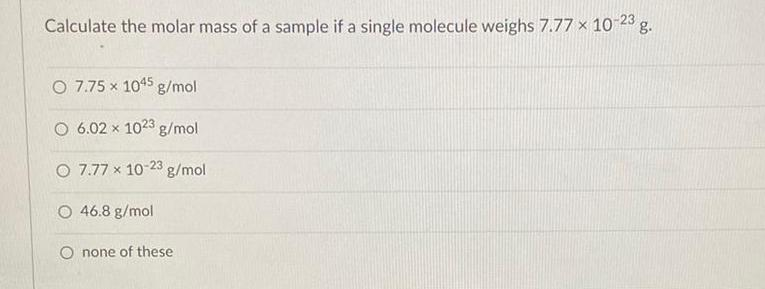Inorganic Chemistry
Preparation and Properties of Compounds
Calculate the molar mass of a sample if a single molecule weighs 7.77 x 10-23 g. 7.75 x 1045 g/mol 6.02 x 1023 g/mol 7.77 x 10-23 g/mol 46.8 g/mol none of these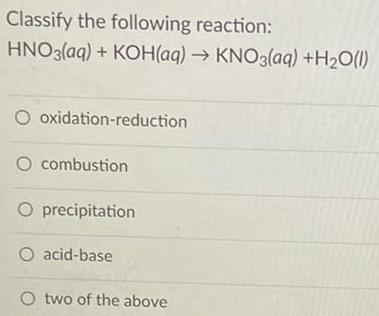Inorganic Chemistry
Preparation and Properties of Compounds
Classify the following reaction: HNO3(aq) + KOH(aq) → KNO3(aq) +H₂O(l) oxidation-reduction combustion precipitation acid-base two of the above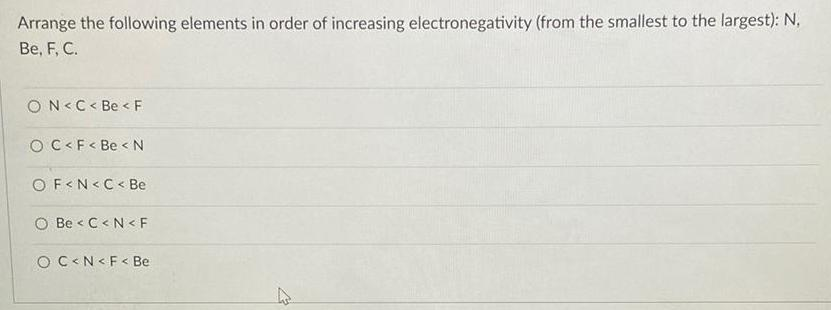Inorganic Chemistry
Preparation and Properties of Compounds
Arrange the following elements in order of increasing electronegativity (from the smallest to the largest): N, Be, F, C. N<C< Be < F C< F< Be <N F<N<C< Be Be <C<N<F C<N< F< Be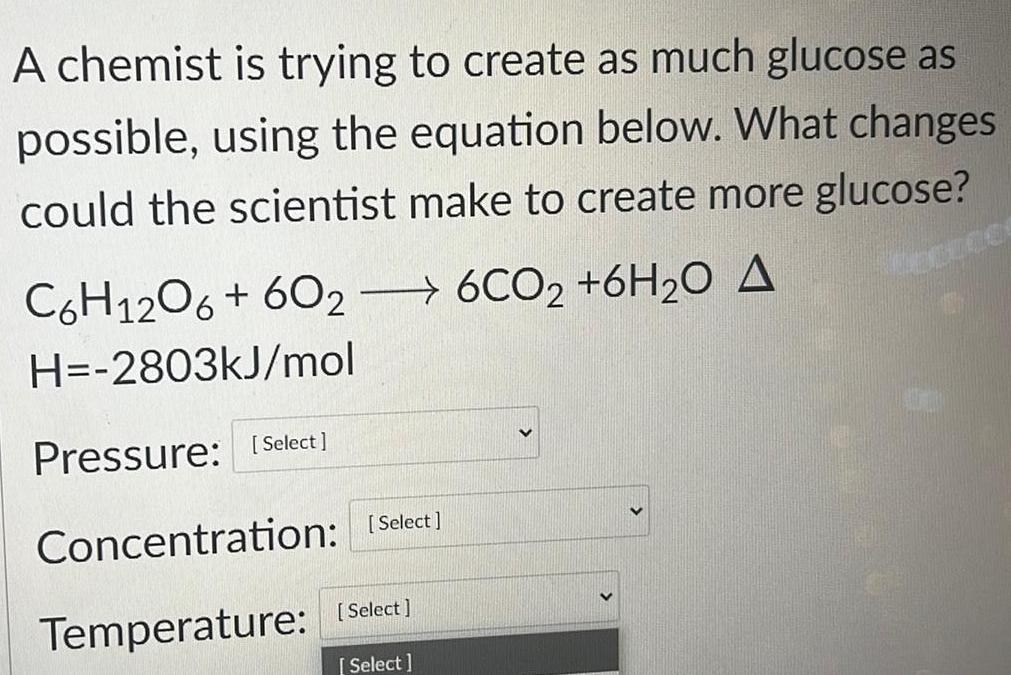Inorganic Chemistry
Preparation and Properties of Compounds
A chemist is trying to create as much glucose as possible, using the equation below. What changes could the scientist make to create more glucose? C6H12O6 + 6026CO2 +6H₂O A H=-2803kJ/mol Pressure: Concentration: Temperature: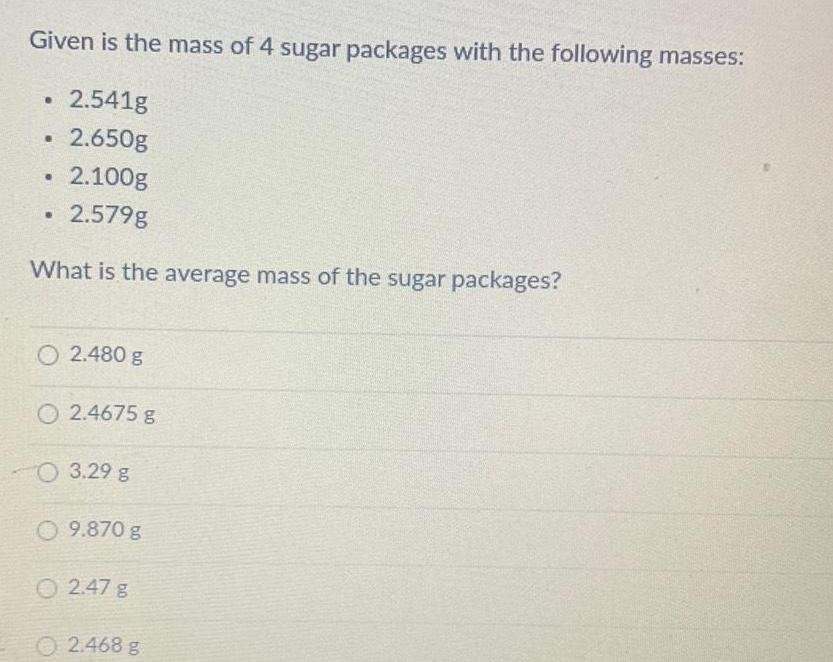Inorganic Chemistry
Preparation and Properties of Compounds
Given is the mass of 4 sugar packages with the following masses: 2.541g 2.650g 2.100g 2.579g What is the average mass of the sugar packages? 2.480 g 2.4675 g 3.29 g 9.870 g 2.47 g 2.468 g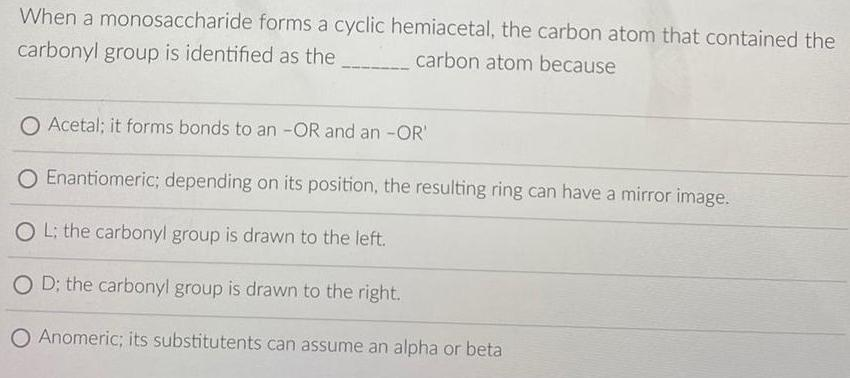Inorganic Chemistry
Preparation and Properties of Compounds
When a monosaccharide forms a cyclic hemiacetal, the carbon atom that contained the carbonyl group is identified as the ___________ carbon atom because Acetal; it forms bonds to an -OR and an -OR' Enantiomeric; depending on its position, the resulting ring can have a mirror image. OL: the carbonyl group is drawn to the left. D; the carbonyl group is drawn to the right. Anomeric; its substitutents can assume an alpha or beta